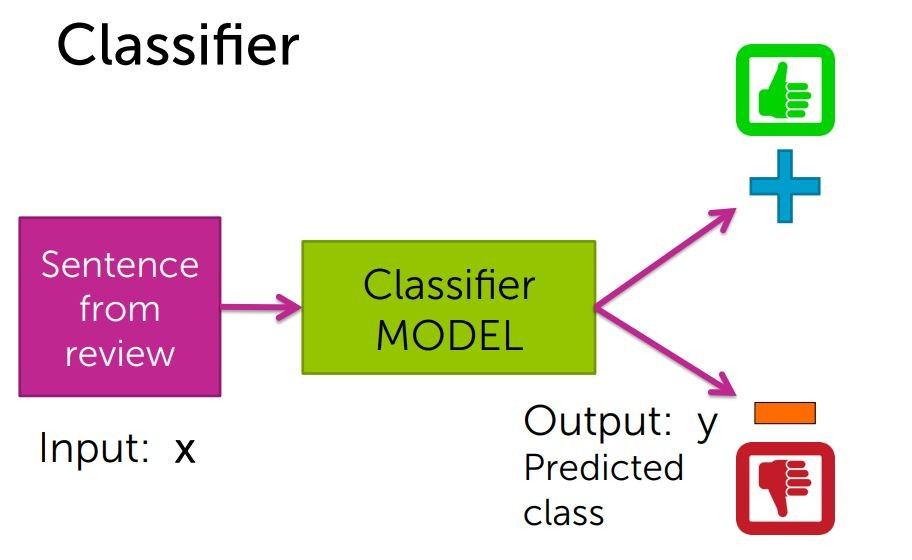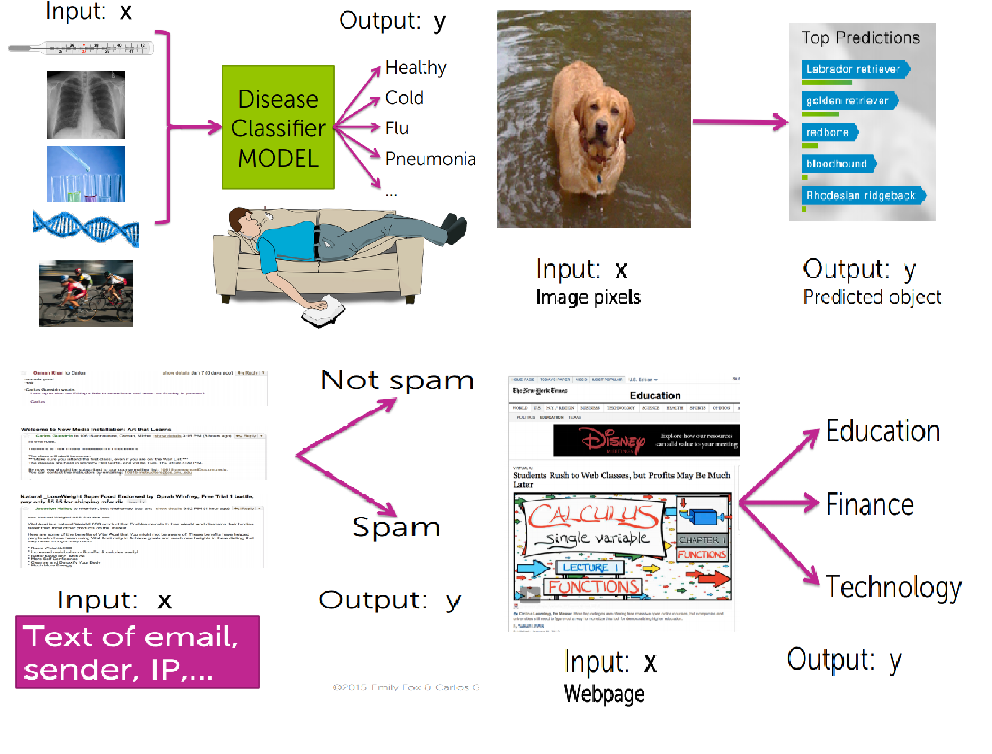2018 iT 邦幫忙鐵人賽DAY 6
0
AI & Machine Learning

ClassifictaionExamples of classification tasks• 醫療診斷除了倚靠顯而易見的體溫、X光、抽血外，現在可以加上DNA序列甚至生活習性來透過分類器診療
• 在影像辨識上透過分類可以更精確是測出狗的品種
• 過濾垃圾郵件，事實上20世紀初的郵件過濾都是手動加上特徵，所以垃圾郵件製作人，也試圖改變某些字眼被過濾掉，它不單單只考慮文字上的特徵，也考慮了發信者的IP位置，發信人是否跟你是熟識的...
• 分類過濾出找出你感興趣的網頁

Linear classifiers

Linear classifiers 是最常見的分類器，那其classifiers mode是如何運作的呢?

input = '涼麵過譽，服務品質普通，味增湯好喝，溫泉蛋不錯吃'
List of positive words = ['不錯','好','棒','讚','驚豔','...']
List of negative words = ['普通','差','難吃','屎','過譽','...']

count positive & negative words in input:
List of positive words in inptu:
positive ++
List of negative words in input:
negative ++

if number of positive words >  number of negative words:
ŷ = +
else:
ŷ = -

-字詞該從那裏取得?
-字詞該怎麼分配權重?(好、棒、讚，那一個好?難吃、屎、過譽，那一個壞?)
-單一個字詞沒辦法分類，像是好吃跟不好吃，其實差了一個字，但評價完全是相反的

Word Weight

1.5

-2.1

-5

input = '涼麵過譽，服務品質普通，味增湯好喝，溫泉蛋不錯吃'

socre(x) = (-3) + (-1) + (1.5) + (0.5) = -2

if score(x) > 0
ŷ = +
esle score(x) <= 0
ŷ = -